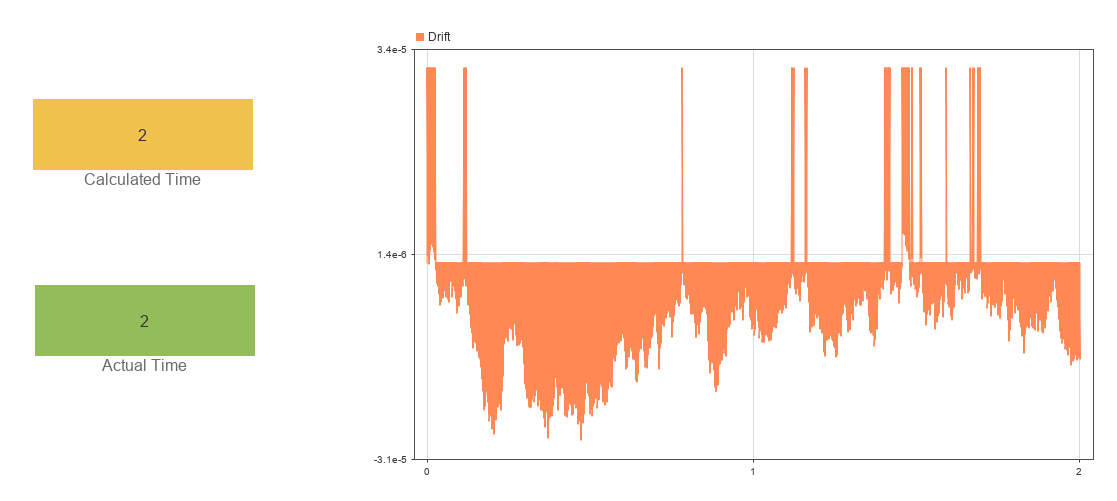# Model Effect of Temperature and Jitter on Crystal Oscillation Frequency

Many digital clocks use a crystal oscillator. The oscillator has a nominal frequency. Based on this frequency, the clock can calculate elapsed time by tracking the crystal oscillations.

The oscillator frequency can vary with environmental conditions such as electronic noise, or jitter, and the ambient temperature. You can use a Hit Scheduler block to model and simulate the effect of time-varying environmental conditions on the oscillation frequency.

### Open and Analyze Model

Open the model `ClockDrift`.

```mdl = "ClockDrift"; open_system(mdl)```The model uses a Signal Editor block to load input data for different ambient temperature scenarios. Based on the current ambient temperature, the `Oscillation Period` subsystem calculates the oscillation period using the equation

$\Delta \mathit{f}={\mathit{f}}_{0}^{}\left(1-\mathit{k}{\left({\mathit{T}}_{0}^{}-{\mathit{T}}_{\mathrm{amb}}^{}\right)}^{2}\right)$,

where:

• $\Delta \mathit{f}$ is the frequency shift due to temperature.

• ${\mathit{f}}_{0}^{}$ is the nominal frequency for the crystal, `32` kHz in this example.

• $\mathit{k}$ is a physical constant that represents the peak temperature drift, `0.04` in this example.

• ${\mathit{T}}_{0}^{}$is the nominal temperature, `25` Celsius in this example.

• ${\mathit{T}}_{\mathrm{amb}}^{}$ is the ambient temperature.

Create a `Simulink.SimulationInput` object that sets the values of ${\mathit{f}}_{0}^{}$, $\mathit{k}$, and ${\mathit{T}}_{0}^{}$for the simulation.

```simIn = Simulink.SimulationInput(mdl); simIn = setVariable(simIn,"f0",32000); simIn = setVariable(simIn,"k",0.04); simIn = setVariable(simIn,"T0",25);```

A Random Number block generates variation in the oscillation period due to noise. The sum of the oscillation period calculated based on the ambient temperature and the variation due to noise represents the delay to the next oscillation. This sum also provides the delay input for the Hit Scheduler block.

The Hit Scheduler block is configured to generate a signal output. On a time step scheduled by the Hit Scheduler block, the output value is `1`, and on other time steps, the value is `0`. The signal output represents the oscillations and provides the enable input for scheduling each time hit.

The model calculates the time for the clock that uses the oscillator by counting the oscillations and multiplying by the nominal period for the oscillator. Due to the temperature variation and jitter, the nominal period is an estimation and the calculated time is not exact. A Clock block provides an indication of the true time for each time step.

### Simulate Drift for High Ambient Temperature

As saved, the Signal Editor block loads data for a constant ambient temperature of 100 C. Simulate the model using this scenario.

`out = sim(simIn);`

Two Display blocks show the calculated and actual time for each time step. The Dashboard Scope block shows the difference between the calculated time and actual time over the duration of the simulation. The drift error accumulates, resulting in a calculated time of `1.9996` seconds for the final simulation time of `2` seconds.### Simulate Drift for Nominal Temperature

To simulate the clock drift when the ambient temperature is `25` C, change the scenario the Signal Editor block loads.

1. Double-click the Signal Editor block.

2. In the Block Parameters dialog box, from the Active scenario list, select `Celsius25`.

3. Click OK.

Alternatively, use the `setBlockParameter` function to modify the block parameter for the `Simulink.SimulationInput` object `simIn`.

`simIn = setBlockParameter(simIn,"ClockDrift/Ambient Temp","ActiveScenario","Celsius25");`

Simulate the model again.

`out = sim(simIn);`

At the nominal temperature, the oscillator frequency has no drift due to temperature. All the drift comes from the system noise, or jitter. For a simulation time of `2` seconds, the jitter in the system has no perceptible effect on the calculated time.